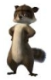# SL4: Binding to generic data

ChartTop achievementsRank 2
Eric Schoenholzer asked on 06 Apr 2010, 11:00 PM

Hi,

I would like to bind a 'generic data' collection to different chart types (via TemplateSelector).

Until now I used as type for the X and Y values a numeric type.

 public class DataPoint { [Key] public Guid Id { get; set; } public int ParentId { get; set; } public int X { get; set; } public int Y { get; set; } public string Entry { get; set; } }

But to be more generic, the X (and Y) axis should accept number, date or string.

I thought to change X and Y property to string, binding it to the chart and setting the FieldType property via XAML to the desired type.

But it seems it's not possible to set the FieldType via XAML because of a SL limitation.

What's the best solution to my problem?

How will chart handle the data when I use always the string type?

Will it detect the correct underlying type?

Or perhaps using a ValueConverter?

Or adding all possible types to the datapoint class? (-> lot of overhead)

I'm using SL4/2010.

Thanks

Eric

## 1 Answer, 1 is accepted

0Giuseppe
Telerik teamanswered on 09 Apr 2010, 02:55 PM
Hello Eric,

• RadChart displays only numerical data so any data that is bound to DataPointMember.YValue should be numerical, too (no strings / dates are supported for Y).
• Item.FieldType will not be taken into account, in case you bind string data to the horizontal axis -- it will always be treated as categorical data and will not be converted.
• If you would like to use "generic" collection, you can define one whose X property type is object (not string), but you would still need to set the proper ItemMapping (DataPointMember.XValue or DataPointMember.XCategory) so the data is interpreted correctly:

The X value interpreted as category data:
``` ```
`public` `class` `ChartData`
`{`
`    ``public` `int` `YValue`
`    ``{`
`        ``get``;`
`        ``set``;`
`    ``}`

`    ``public` `object` `XValue`
`    ``{`
`        ``get``;`
`        ``set``;`
`    ``}`
`}`

`public` `partial` `class` `MainPage : UserControl`
`{`
`    ``public` `MainPage()`
`    ``{`
`        ``InitializeComponent();`

`        ``List<ChartData> data = ``new` `List<ChartData>();`
`        ``data.Add(``new` `ChartData() { XValue = ``"test"``, YValue = 12 });`
`        ``data.Add(``new` `ChartData() { XValue = ``"test2"``, YValue = 52 });`
`        ``data.Add(``new` `ChartData() { XValue = ``"test3"``, YValue = 32 });`
`        ``data.Add(``new` `ChartData() { XValue = ``"test4"``, YValue = 22 });`

`        ``SeriesMapping sm = ``new` `SeriesMapping();`
`        ``sm.ItemMappings.Add(``new` `ItemMapping(``"XValue"``, DataPointMember.XCategory));`
`        ``sm.ItemMappings.Add(``new` `ItemMapping(``"YValue"``, DataPointMember.YValue));`

`        ``RadChart1.SeriesMappings.Add(sm);`
`        ``RadChart1.ItemsSource = data;`
`    ``}`
`}`

The X value interpreted as DateTime data:
`public` `class` `ChartData`
`{`
`    ``public` `int` `YValue`
`    ``{`
`        ``get``;`
`        ``set``;`
`    ``}`

`    ``public` `object` `XValue`
`    ``{`
`        ``get``;`
`        ``set``;`
`    ``}`
`}`

`public` `partial` `class` `MainPage : UserControl`
`{`
`    ``public` `MainPage()`
`    ``{`
`        ``InitializeComponent();`

`        ``List<ChartData> data = ``new` `List<ChartData>();`
`        ``data.Add(``new` `ChartData() { XValue = DateTime.Today, YValue = 12 });`
`        ``data.Add(``new` `ChartData() { XValue = DateTime.Today.AddDays(1), YValue = 52 });`
`        ``data.Add(``new` `ChartData() { XValue = DateTime.Today.AddDays(5), YValue = 32 });`
`        ``data.Add(``new` `ChartData() { XValue = DateTime.Today.AddDays(7), YValue = 22 });`

`        ``SeriesMapping sm = ``new` `SeriesMapping();`
`        ``sm.ItemMappings.Add(``new` `ItemMapping(``"XValue"``, DataPointMember.XValue));`
`        ``sm.ItemMappings.Add(``new` `ItemMapping(``"YValue"``, DataPointMember.YValue));`

`        ``RadChart1.SeriesMappings.Add(sm);`
`        ``RadChart1.ItemsSource = data;`
`    ``}`
`}`

Hope this helps.

Regards,
Freddie
the Telerik team

Do you want to have your say when we set our development plans? Do you want to know when a feature you care about is added or when a bug fixed? Explore the Telerik Public Issue Tracking system and vote to affect the priority of the items.
Tags
ChartRank 2